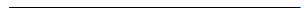Search:

# The buffers

## Definition

A buffer is a mixture solution of a weak acid with its corresponding base provided that: $3\;\lt\; pK_a\;\lt\;11$

Examples: $NH_4^+/NH_3$ (in the urine) $H_2O+CO_2/HCO_3^-$ (in the blood)

## pH of buffers

The trivial equilibrium: $HB$ $+$ $B$ $\rightleftarrows$ $B$ $+$ $HB$ does not change the initial concentrations and reactions of both species $HB$ and $B$ with water only provide low and comparable amounts of $B$ by $HB$, so we can say: $c_{HB}$ $\approx$ $[HB]$ $c_{B}$ $\approx$ $[B]$, thus: $[H_3O^+]$ $=$ $K_a\frac{[HB]}{[B]}$ $\approx$ (we will write later = ) $K_a\frac{c_{HB}}{c_B}$

$pH$ of a buffer: $pH$ $=$ $pK_a$ $+$ $log\frac{c_B}{c_{HB}}$

If $n_{HB}$ and $n_{B}$ are the initial number of moles : $pH$ $=$ $pK_a$ $+$ $log\frac{c_B}{c_{HB}}$ = $pK_a$ $+$ $log\frac{\frac{n_B}{V}}{\frac{n_{HB}}{V}}$

$pH$ of a buffer: $pH$ $=$ $pK_a$ $+$ $log\frac{n_B}{n_{HB}}$

Example 1: $1\;mol$ $CH_3COOH$ and $1\;mol$ $CH_3COONa$ are diluted together to produce $1\;L$ of buffer solution: $pH$ $=$ $pK_a$ $+$ $log\frac{n_B}{n_{HB}}$ = $4.75$ $+$ $log\frac{1}{1}$ = $4.75$

Example 2: $100\; mL$ $CH_3COOH$ $0.1\; M$ are mixed with $50\; mL$ $CH_3COONa$ $0.4\; M$ to form a buffer: $c_{CH_3COOH}$ $=$ $\frac{0.1\cdot 0.1}{0.15}$ $c_{CH_3COO^-}$ $=$ $\frac{0.05\cdot 0.4}{0.15}$ $pH$ $=$ $pK_a$ $+$ $log\frac{c_{CH_3COO^-}}{c_{CH_3COOH}}$ = $5.05$

## Buffers and dilution

Diluting a buffer does not change the number of moles of acid or the number of moles of base, thus: $pK_a$ $=$ $pH + log\frac {n_B}{n_HB}$

The dilution does not affect the pH of a buffer

## Buffers and the addition of acids or bases

Consider for example (1) $900\;mL$ buffer containing $0.1\;mol$ and $0.1\;mol$ ethanoic ethanoate, $pH$ is 4.75 (2) $900\; mL$ of pure water, the $pH$ is 7Add $100\; mL$ of hydrochloric acid containing $0.01\;mol$ $HCl$: - By adding to (1), we obtain $0.11\;mol$ of ethanoic acid, there are still $0.09\;mol$ ethanoate, the $pH$ will be 4.66 The $pH$ decreased from $4.75$ to $4.66$ = 0.09 - By adding to (2), there are still $0.01\; mol$ $HCl$ in $1\; L$ of solution, the $pH$ will be equal to 2 The $pH$ decreased $7-2 =$ 5Add $100\; mL$ of sodium hydroxide containing $0.01\; mol$ NaOH : - By adding to (1), there remain $0.09\; mol$ of ethanoic acid, we obtain $0.11\; mol$ ethanoate, the $pH$ will be 4.84 The $pH$ increased from $4.84$ to $4.75$ = 0.09 - By adding to (2), there will be $0.01\; mol$ $NaOH$ in $1 \;L$ of solution, the $pH$ will be equal to 12 The $pH$ increased from $12-7$ = 5

The above examples suggest that

A buffer "buffers"(= amortizes) $pH$ variations